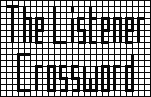## Listener Crossword 3970: Solution NotesBack to 2008 menu

## Beating the Bookies by Ruslan

### Puzzle explanation

As explained in the working below, the odds are: Agio 20/1, Ciao 4/1, Ergo 2/1, Filo 8/1, Iago 33/1, Lido 100/1, Mojo 60/1, Nemo 11/1, Olio 16/1, Sumo 15/1, Tyro 6/1, Xeno 7/1.

The smallest profit occurs when the pay-out equals the bet but is rounded up, producing a 1p profit: this can happen only when two of the odds are odd and the third is even. The only possibility is for the winner to be 8/1 (Filo) and the places 7/1 (Xeno) and 33/1 (Iago). The ordering of the places is determined by the initials spelling FIX.

If S is the stake and x to 1 the odds, payouts are

• on win bet, S(1+x),
• on e/w bet (win), S(2+1.25x),
• on e/w bet (place), S(1+.25x).
Bets to win that are multiples of 10p correspond to entries ending in 0: this applies to 1a, 8a and 2d, 14d and 18a.

18a has to be 50 and C is 4/1, so 1d is 125.

8a is 510 (16/1), 540 (17/1) or 570 (18/1). 13d is 2200 (16/1), 2325 (17/1) or 2450 (18/1).

12d must start with a 1 so 12a is 1x2. Possibilities are 105 (8/1), 114 (9/1), 123 (10/1), 132 (11/1), 140 (12/1) ... 175 (16/1), 184 (17/1), 193 (18/1), so N is 11/1, 12a is 132 and 4d is 1103.

1a has A > 13/1, 15d has A < 22/1.

1a is 1050 (14/1), 1120 (15/1), 1190 (16/1), 1260 (17/1), 1330 (18/1), 1400 impossible (2d starts 0), 1470 (20/1) or 1540 (21/1).

2d ends in 0 so S odds are odd. 2d has S < 22/1.

3a is x1x and S > 13/1, so S is 15/1, 3a is 119 and 2d is 720, making 1a 1470 and A 20/1. 15d is 945.

17a is xx4x.

12d has L > 68.

17a is 1643 (69/1), 1845 (78/1), 2048 (87/1) or 2340 (100/1).

12d is 1004 (69/1), 1128 (78/1), 1252 (87/1) or 1430 (100/1). So L is 100/1 and 6a is 2210. 3d is 100 so T is 6/1. 14d is 140.

19a is 105 (2/1) or 805 (42/1). 10d is 15 (2/1) or 115 (42/1) so E is 2/1, 19a is 105 and 10d 15.

5d has 58/1 < M < 66/1. 9a is 5005 so M is 60/1 and 5d is 915.

7d is 152 so X is 7/1 and 10a is 1022.

6d is 210 (8/1) or 280 (12/1), but as 8a is 510 (16/1), 540 (17/1) or 570 (18/1), F is 8/1, O is 16/1, 6d is 210 and 8a is 510. 13d is 2200.

14a is 1xx4, either 1164 (25/1), 1514 (33/1) or 1864 (41/1).

11d is 1995 (25/1), 2595 (33/1) or 3195 (41/1). So I is 33/1, 14a is 1514, 11d is 2595 and 16a is 649.

If x1, x2 and x3 are the odds of the first three horses, the smallest profit could occur if the pay out equalled the total bet but was rounded up to give a 1p profit, ie, if 12 = 2 + 0.5 x1 +0.5(0.25 x1 + 0.25 x2 + 0.25 x3), 80 - 5 x1 = x2 + x3.

This is only true when x1 =8, x2 and x3 are 7 and 33.

8/1 winner pays Ł4.50, 8/1 place pays Ł1.50, 7/1 place Ł1.38 and 33/1 place Ł 4.63, total Ł12.01.

Filo wins, Iago is second and Xeno third — spelling FIX.

Back to 2008 menu